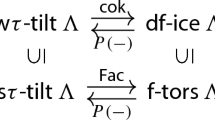# Wide Subcategories and Lattices of Torsion Classes

## Abstract

In this paper, we study the relationship between wide subcategories and torsion classes of an abelian length category $$\mathcal {A}$$ from the point of view of lattice theory. Motivated by τ-tilting reduction of Jasso, we mainly focus on intervals $$[\mathcal {U},\mathcal {T}]$$ in the lattice $$\operatorname {\mathsf {tors}} \mathcal {A}$$ of torsion classes in $$\mathcal {A}$$ such that $$\mathcal {W}:=\mathcal {U}^{\perp } \cap \mathcal {T}$$ is a wide subcategory of $$\mathcal {A}$$; we call these intervals wide intervals. We prove that a wide interval $$[\mathcal {U},\mathcal {T}]$$ is isomorphic to the lattice $$\operatorname {\mathsf {tors}} \mathcal {W}$$ of torsion classes in the abelian category $$\mathcal {W}$$. We also characterize wide intervals in two ways: First, in purely lattice theoretic terms based on the brick labeling established by Demonet–Iyama–Reading–Reiten–Thomas; and second, in terms of the Ingalls–Thomas correspondences between torsion classes and wide subcategories, which were further developed by Marks–Šťovíček.

This is a preview of subscription content, log in via an institution to check access.

Price excludes VAT (USA)
Tax calculation will be finalised during checkout.

### Similar content being viewed by others### ICE-closed subcategories and wide $$\tau$$ -tilting modules

Haruhisa Enomoto & Arashi Sakai

### Torsion theories connected by a heart and hearts of intervals

Takeshi Yoshizawa

Haruhisa Enomoto

## References

1. Adachi, T., Iyama, O., Reiten, I.: τ-tilting theory. Compos. Math. 150(3), 415–452 (2014)

2. Asai, S.: Semibricks, Int. Math. Res. Not. IMRN. 16, 4993–5054 (2020)

3. Asai, S.: The wall-chamber structures of the real Grothendieck groups. Adv. Math. 381, 107615 (2021)

4. Barnard, E., Carroll, A., Zhu, S.: Minimal inclusions of torsion classes. Algebr. Comb. 2(5), 879–901 (2019)

5. Baumann, P., Kamnitzer, J., Tingley, P.: Affine Mirković-Vilonen polytopes. Publ. Math. Inst. Hautes Études Sci. 120, 113–205 (2014)

6. Brenner, S., Butler, M. C. R.: Generalizations of the Bernšteı̆n–Gel’fand–Ponomarev reflection functors, Representation theory, II (Proc. Second Internat. Conf., Carleton Univ., Ottawa, Ont., 1979), 103–169 Lecture Notes in Math, vol. 832. Springer, Berlin (1980)

7. Bridgeland, T.: Stability conditions on triangulated categories. Ann. Math. (2) 166(2), 317–345 (2007)

8. Bridgeland, T.: Scattering diagrams, Hall algebras and stability conditions. Algebr. Geom. 4(5), 523–561 (2017)

9. Brüning, K.: Thick subcategories of the derived category of a hereditary algebra. Homology Homotopy Appl. 9(2), 165–176 (2007)

10. Brüstle, T., Smith, D., Treffinger, H.: Wall and Chamber Structure for finite-dimensional Algebras. Adv. Math. 354, 106746 (2019)

11. Brüstle, T., Yang, D.: Ordered exchange graphs, Advances in representation theory of algebras, pp. 135–193, EMS Ser. Congr. Rep., Eur. Math. Soc. Zürich (2013)

12. Demonet, L., Iyama, O., Jasso, G.: τ-tilting finite algebras, bricks, and g-vectors. Int. Math. Res. Not. IMRN. (3), 852–892 (2019)

13. Demonet, L. , Iyama, O., Reading, N., Reiten, I., Thomas, H.: Lattice theory of torsion classes, arXiv:1711.01785v2

14. Dickson, S. E.: A torsion theory for Abelian categories. Trans. Amer. Math. Soc. 121(1), 223–235 (1966)

15. Happel, D.: Triangulated categories in the representation theory of finite dimensional algebras. London Mathematical Society Lecture Note Series, vol. 119. Cambridge University Press (1988)

16. Happel, D., Reiten, I., Smalø, S. O.: Tilting in abelian categories and quasitilted algebras. Mem. Amer. Math. Soc. 120(575) (1996)

17. Hovey, M.: Classifying subcategories of modules. Trans. Amer. Math. Soc. 353(8), 3181–3191 (2001)

18. Ingalls, C., Thomas, H.: Noncrossing partitions and representations of quivers. Compos. Math. 145(6), 1533–1562 (2009)

19. Iyama, O., Reading, N., Reiten, I., Thomas, H.: Lattice structure of Weyl groups via representation theory of preprojective algebras. Compos. Math. 154(6), 1269–1305 (2018)

20. Jasso, G.: Reduction of τ-tilting modules and torsion pairs. Int. Math. Res. Not. IMRN 16, 7190–7237 (2015)

21. King, A. D.: Moduli of representations of finite dimensional algebras. Quart. J. Math. Oxford Ser. (2) 45(180), 515–530 (1994)

22. Koenig, S., Yang, D.: Silting objects, simple-minded collections, t-structures and co-t-structures for finite-dimensional algebras. Doc. Math. 19, 403–438 (2014)

23. Marks, F., Šťovíček, J.: Torsion classes, wide subcategories and localisations. Bull. Lond. Math. Soc. 49(3), 405–416 (2017)

24. Mizuno, Y.: Classifying τ-tilting modules over preprojective algebras of Dynkin type. Math. Z. 277(3-4), 665–690 (2014)

25. Rickard, J.: Morita theory for derived categories. J. Lond. Math. Soc. (2) 39(3), 436–456 (1989)

26. Ringel, C. M.: Representations of K-species and bimodules. J. Algebra 41(2), 269–302 (1976)

27. Yurikusa, T.: Wide Subcategories are Semistable. Doc. Math. 23, 35–47 (2018)

## Acknowledgements

The authors thank Aaron Chan, Laurent Demonet, Haruhisa Enomoto, Osamu Iyama, Gustavo Jasso and Jan Schröer for kind instructions and discussions. The second named author would like to thank the Mathematical Institute of the University of Bonn, where most of his work was done as part of his Master studies.

## Funding

The first named author was supported by Japan Society for the Promotion of Science KAKENHI JP16J02249, JP19K14500 and JP20J00088.

## Author information

Authors

### Corresponding author

Correspondence to Sota Asai.

Presented by: Henning Krause

### Data sharing statement

Data sharing is not applicable to this article as no datasets were generated or analysed during the current study.

### Publisher’s Note

Springer Nature remains neutral with regard to jurisdictional claims in published maps and institutional affiliations.

## Rights and permissions

Reprints and Permissions